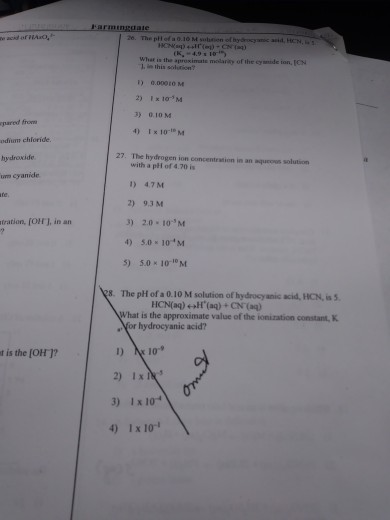# Question & Answer: The pH of a 0.10 M solution of hydrocyanic acid, HCN HCr (aq) doubleheadarrow H (aq) + CN…..mult choice 26 and 27

Don't use plagiarized sources. Get Your Custom Essay on
Question & Answer: The pH of a 0.10 M solution of hydrocyanic acid, HCN HCr (aq) doubleheadarrow H (aq) + CN…..
GET AN ESSAY WRITTEN FOR YOU FROM AS LOW AS \$13/PAGE

The pH of a 0.10 M solution of hydrocyanic acid, HCN HCr (aq) doubleheadarrow H (aq) + CN (aq) (K_ = -4.9 times 10^ What is the approximate morality of the cyanide ion, [CN in this solution? 1) 0.00010 M 2) 1 times 10^-10 M 3) 0.10 M 4) 1 times 10^-10 M The hydrogen ion concentration in an aqueous solution with a pH of 4.70 is 1) 4.7M 2) 9.3 M 3) 2.0 10^-5 M 4) 5.0 10^-4 M 5) 5.0 times 10^-10 M The pH of a 0.10 M solution of hydrocyanic acid, HCN, is 5. HCN(aq) doubleheadarrow H^ (aq) + CN^ (aq) What is the approximate value of the ionization constant, for hydrocyanic acid? 1) 1 times 10^-9 2) 1 times 10^-5 c) 1 times 10^-4 4) 1 times 10^-1

26.

HCN(aq) <—-> H^+(aq) + CN^-(aq)

if pH = 5

[H3O+] = 10^-pH

= 10^-5

from equation [H+] = [CN-] = 1*10^-5 M